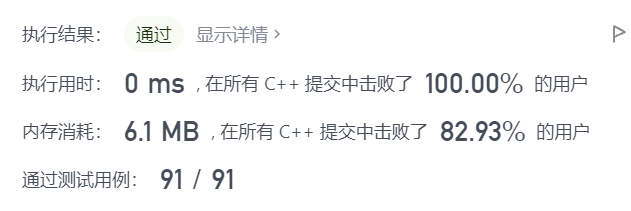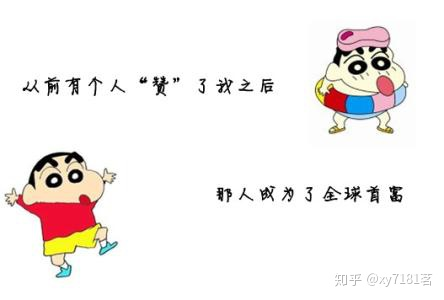• 请编写一个程序检查表达式中的左右圆括号是否匹配，若匹配，则返回“YES”；否则返回“NO”。表达式长度小于255，左圆括号少于20个。 输入格式 一行：表达式 输出格式 一行：“YES” 或“NO” 输入输出样例 ...

题目描述

假设一个表达式有英文字母（小写）、运算符（+，—，*，/）和左右小（圆）括号构成，以“@”作为表达式的结束符。请编写一个程序检查表达式中的左右圆括号是否匹配，若匹配，则返回“YES”；否则返回“NO”。表达式长度小于255，左圆括号少于20个。

一行：表达式

输出格式

一行：“YES” 或“NO”

输入输出样例

输入 #1：2*(x+y)/(1-x)@

输出 #1：YES

输入 #2：(25+x)*(a*(a+b+b)@

输出 #2：NO

说明/提示

表达式长度小于255，左圆括号少于20个

#include <bits/stdc++.h>
using namespace std;
int main()
{
string s;
int num=0;
cin>>s;
int len=s.length();
for(int i=0;i<len;i++)
{
if(num<0)
{
cout<<"NO";
return 0;
}
if(s[i]=='(') num++;
if(s[i]==')') num--;
}
if(num==0) cout<<"YES";
else cout<<"NO";
}

展开全文c++
• C++实现一个简单的括号匹配

千次阅读 2021-04-25 11:24:33
如下所示，这是一个简单的括号匹配： #include<iostream> #include<cstring> using namespace std; class stack { private: int maxsize; char * st; public: int top; stack(int size) { ...

如下所示，这是一个简单的括号匹配：

#include<iostream>
#include<cstring>
using namespace std;
class stack
{
private:
int maxsize;
char * st;
public:
int top;
stack(int size)
{
maxsize=size;
top=-1;
st=new char[maxsize];
}
void push(char num)
{
if(top==maxsize-1)
{
cout<<"栈满溢出"<<endl;
}
else
{
st[++top]=num;
}
}
char pop()
{
char num;
if(top==-1)
{
//cout<<"栈为空，不能删除"<<endl;
return 0;
}
else
{
num=st[top--];
return num;
}
}
};
int main()
{
int i;
stack s1(10),s2(10),s3(10);
char c;
cin>>c;
int len=strlen(c);
for(i=0;i<len;i++)
{
if(c[i]=='(')
s1.push(c[i]);
if(c[i]=='{')
s2.push(c[i]);
if(c[i]=='[')
s3.push(c[i]);
if(c[i]==')')
{
if(s1.top!=-1)
{
if(s1.pop()=='(')
cout<<"()配对成功"<<endl;
}
else
cout<<"()匹配失败"<<endl;
}
if(c[i]=='}')
{
if(s2.top!=-1)
{
if(s2.pop()=='{')
cout<<"{}配对成功"<<endl;
}
else
cout<<"{}匹配失败"<<endl;
}
if(c[i]==']')
{
if(s3.top!=-1)
{
if(s3.pop()=='[')
cout<<"[]配对成功"<<endl;
}
else
cout<<"[]匹配失败"<<endl;
}
}
if(s1.top!=-1)
cout<<"[]匹配失败"<<endl;
if(s2.top!=-1)
cout<<"[]匹配失败"<<endl;
if(s3.top!=-1)
cout<<"[]匹配失败"<<endl;
return 0;
}

展开全文算法 stack
• 20. 有效的括号（力扣） 给定一个只包括 '('，')'，'{'，'}'，'['，']'的字符串 s ，判断字符串是否有效。 有效字符串需满足： 左括号必须用相同类型的右括号闭合。 左括号必须以正确的顺序闭合。 示例 1： ...

20. 有效的括号（力扣）

给定一个只包括 '('，')'，'{'，'}'，'['，']' 的字符串 s ，判断字符串是否有效。

有效字符串需满足：

左括号必须用相同类型的右括号闭合。
左括号必须以正确的顺序闭合。

示例 1：

输入：s = "()"
输出：true
示例 2：

输入：s = "()[]{}"
输出：true
示例 3：

输入：s = "(]"
输出：false
示例 4：

输入：s = "([)]"
输出：false
示例 5：

输入：s = "{[]}"
输出：true

来源：力扣（LeetCode）
链接：https://leetcode-cn.com/problems/valid-parentheses

思路一（利用栈）

对字符串从左到右进行处理

如果是左括号（包括 ( , [ , {  ），则入栈；

如果是右括号，则判断栈顶元素是否与右括号匹配

1. 栈空，右括号无法匹配--则直接可以得到结果--false
2. 栈顶元素与右括号不匹配--则直接可以得到结果--false
3. 栈顶元素与右括号匹配--则弹出栈顶元素

处理完字符串后，再判断栈是否为空

• 栈空--说明字符串中的左右括号刚好匹配
• 栈不空--说明左括号有剩余

class Solution {
public:
bool isValid(string s) {
stack<char> v;
for (int i = 0; i < s.size(); i++)
{
if (s[i] == '(' || s[i] == '[' || s[i] == '{')
{
v.push(s[i]);
}
else if (s[i] == ')' || s[i] == ']' || s[i] == '}')
{

if (v.empty()) { return false; }
switch (s[i])
{
case ')':
{
if (v.top() == '(')
{
v.pop();
}
else
return false;
break;
}
case ']':
{
if (v.top() == '[')
{
v.pop();
}
else
return false;
break;
}
case '}':
{
if (v.top() == '{')
{
v.pop();
}
else
return false;
break;
}
}
}

}

if (v.empty())
return true;
else
return false;
}
};问题描述

现在，有一行括号序列，请你检查这行括号是否配对。

第一行输入一个数N

输出

每组输入数据的输出占一行，如果该字符串中所含的括号是配对的，则输出Yes,如果不配对则输出No

3
[(])
(])
([[]()])

No
No
Yes

解法二（利用数组）

感觉我好像写复杂了

#include<iostream>
#include<string>
#include<cstring>
using namespace std;
bool f(char a, char b)
{
if (( a == '(' && b == ')' )|| (a == '{' && b == '}') || ( a == '[' && b == ']'))
return true;
else
return false;
}
int main()
{
char ch;
int n;
cin >> n;
while (n--)
{
ch = '1';
cin >> &ch;
int len = strlen(ch);
for (int i = 2; i <=len; i++)
{
while(f(ch[i-1],ch[i])==true)
{
for (int j = i - 1; j <=len - 1; j++)  //括号匹配时--覆盖匹配的元素
{
ch[j] = ch[j + 1];
}
ch[len-1] = '\0';  //处理字符串结尾
len--;             //数组长度减小
if (i == 0)
{
cout << "YES" << endl;
break;
}
for (int j = i - 1; j <=len - 1; j++)
{
ch[j] = ch[j + 1];
}
ch[len-1] = '\0';   //处理字符串结尾
len--;
i--;
}
}
if (strlen(ch) == 1)
cout << "Yes" << endl;
else
cout << "No" << endl;
}
return 0;
}

展开全文leetcode 算法 c++
• 请编写一个程序检查表达式中的左右圆括号是否匹配，若匹配，则返回“YESYES”；否则返回“NONO”。表达式长度小于255255，左圆括号少于2020个。 【输入】 一行数据，即表达式。 【输出】 一行，即“YESYES”...

【题目描述】

假设一个表达式有英文字母（小写）、运算符（+，—，∗，/）和左右小（圆）括号构成，以“@”作为表达式的结束符。请编写一个程序检查表达式中的左右圆括号是否匹配，若匹配，则返回“YES”；否则返回“NO”。表达式长度小于255，左圆括号少于20个。

一行数据，即表达式。

【输出】

一行，即“YES” 或“NO”。

【输入样例】

2*(x+y)/(1-x)@

YES

【提示】

【样例输入2】

(25+x)*(a*(a+b+b)@

【样例输出2】

NO

代码（第一种方法）：

【思路点拨】

统计有多少个圆括号只有‘（’，如果一有‘）’那么‘（’就有了另一边，直接把统计的‘（’的数量减一，最后判断是不是没有单个的‘（’或‘）’。

#include<bits/stdc++.h>
using namespace std;
int main(){
char a;
int n=0;
cin>>a;
int i=0;
while(true){
if(a[i]=='@')break;
if(a[i]=='(')n++;
if(a[i]==')')n--;
i++;
}
if(n==0){
cout<<"YES";
}else{
cout<<"NO";
}
}//既然看了我的代码，就点个赞呗（不多，就一个赞）！！！

【代码】（第二种方法）：

【思路点拨】

把所有的‘（’统计起来放进栈n里，再把‘）’统计起来放进栈m里，比较两个栈的元素的数量是否相等，简单的说就是‘（’的数量与‘）’的数量是否相等。

#include<bits/stdc++.h>
using namespace std;
int main(){
stack<char> n,m;
char a,b;
int c=0,d,i=0;
cin>>a;
while(1){
if(a[i]=='@')break;
if(a[i]=='(')n.push(a[i]);
if(a[i]==')')m.push(a[i]);
i++;
}
c=n.size();
d=m.size();
if(c!=d){
cout<<"NO";
}else{
cout<<"YES";
}
}//既然看了我的代码，就点个赞呗（不多，就一个赞）！！！展开全文c++
• 括号匹配问题C++ 利用stl库 1. 题目 利用栈来判断括号是否匹配，不限次数地输入，匹配则输出“True”，不匹配就输出“False”。 2. 解析 碰上左边括号压入栈，碰上右边括号则判断： a. 此时栈内是否有元素（注意...c++
• 思路就是对一个字符串分解成一个个字符，然后遍历这个字符串中的每一个字符，如果是左括号，就让他入栈，如果碰到了右括号，就将栈中的括号弹出，看是否与其匹配，若匹配就继续遍历，若不匹配就直接退出，输出false,...c++
• 给定一串字符，不超过100个字符，可能包括括号、数字、字母、标点符号、空格，编程检查这一串字符中的( ) ,[ ],{ }是否匹配。输入格式:输入在一行中给出一行字符串，不超过100个字符，可能包括括号、数字、字母、...
• 给定一串字符（以’#’结束，不超过100个字符），可能包括三种括号：小括号、中括号和大括号，各种括号之间允许任意的嵌套，编程检查这一串字符中的( ) ,[ ],{ }是否匹配。 输入格式: 输入一字符串（以’#’结束，不...c++ 开发语言 数据结构
• 输入一串括号字符串，从左往右扫描，若遇到左括号则入栈，若遇到右括号则从栈顶出栈一个括号(肯定是左括号),然后与其匹配。 代码实现 #include<iostream> #include<string> using namespace std; const ...
• 规定（与常见的算数式子一样）任何一个左括号都从内到外与在它右边且距离最近的右括号匹配。写一个程序，找到无法匹配的左括号和右括号，输出原来字符串，并在下一行标出不能匹配的括号。不能匹配的左括号用"\$“标注...
•算法 c++ 数据结构
• 现在，有一行括号序列，请你检查这行括号是否配对。输入第一行输入一个数N(0输出每组输入数据的输出占一行，如果该字符串中所含的括号是配对的，则输出Yes,如果不配对则输出No样例输入3[(])(])([[]()])样例输出NoNo...
• 检查一段C语言代码的小括号( )、 中括号[ ]和大括号{ }是否匹配 输入格式: 在一行中输入一段C语言代码，长度不超过1000个字符（行末以换行符结束）。 输出格式: 第一行输出左括号的数量和右括号的数量，中间以一...数据结构 c++
• 编写一个C++程式,解决括号匹配问题,即匹配一个字串中的左右括号.以下文字资料是由(历史新知网www.lishixinzhi.com)小编为大家搜集整理后发布的内容，让我们赶快一起来看一下吧！编写一个C++程式,解决括号匹配问题,即...
• 括号则弹出栈顶元素进行匹配 3、匹配成功则继续step2，失败则输出“error” 4、直到遇到“#”结束 5、如果栈循环结束后为空，表示全部匹配成功，输出“true”，否则“error” #include <bits/stdc++.h> #...数据结构 c++
• 括号匹配问题

2021-01-26 23:17:41
给定一个只包括 ‘(’，’)’，’{’，’}’，’[’，’]’ 的字符串，判断字符串是否有效。 有效字符串需满足： ...②如果遇到右括号，则与栈顶元素进行匹配匹配成功则栈顶元素出栈 若匹配失败则返回
• 合法的括号序列称为匹配序列，不合法的括号序列称为不匹配序列。匹配序列示例：（[()]） [] ()[()] ，不匹配序列示例：（[()] ] (][()]，那么如何判断一个括号序列是否为匹配序列呢？请用栈的结构来进行验证。 分析 ...c++ 数据结构
• 一、问题描述： 设计算法检测表达式的括号是否匹配 注：包括 () [] {} 二、解题思路： 栈的应用 例如： ...当遇到右括号 )]} 时，将栈中的字符出栈并与此右括号比较，若此括号匹配，即 当右括号为数据结构 算法
• 括号匹配深度：c++ 一个合法的括号匹配序列有以下定义: 1、空串"“是一个合法的括号匹配序列 2、如果"X"和"Y"都是合法的括号匹配序列,“XY"也是一个合法的括号匹配序列 3、如果"X"是一个合法的括号匹配序列,那么”(X...c++
• C++括号匹配

2021-09-24 15:20:50
一个括号匹配方法，用到数据结构栈 #include <string> #include <iostream> #include <stack> #include <string> using namespace std; string::iterator it; stack<char>st; bool ...c++ 数据结构
• 括号匹配问题简单括号匹配问题是给出字符串，判断字符串中的括号是否匹配，此类问题核心解决方案就是利用栈的后进先出的特性，从左到右依次遍历字符串，遇左括号进栈，遇右括号将其与栈顶元素配对，若能配对，则栈顶...
• } if (str[i] == ')' || str[i] == ']' || str[i] == '}') { if (top == NULL){ cout 括号匹配!" ; break; } else { Node* pt = top; top = top->next; pt->next = NULL; char item = pt->ch; delete pt; if (...
• 系列文章目录 ...使用栈判断字符串中的括号是否匹配 二、c++代码 1.顺序栈 代码如下： #include<iostream> using namespace std; #define MaxSize 100 typedef char datatype; typedef struct {
• 括号匹配实验报告书1．实验题目括号匹配的检验2．需求分析圆括号方括号，其嵌套的顺序随意，即(()[ ])或([ ] [ ])等为正确格式，[[ )]或((()均为不正确的格式。输入([ ]())，结果“匹配”输入 [( [)]，结果“此串...
• 继续遍历字符串，当遇到右括号且栈非空，则括号匹配数加一 3.继续循环遍历，如果遇到不是 ( 或 ）时，遍历下个字符 函数代码： void Question3(char str[]) { char* p = NULL; p = str; stacks; int count = 0; ...c++
• } else { if (IsEmtpy(S)) { //若扫描到右括号 判空（如果空则表示没有和左括号匹配的），失败 return false; } char topElem; Pop(S, topElem); //存储出栈的元素 if (str[i]==')'&&...c++ 开发语言 后端
• 本文主要向大家介绍了C/C++知识点之C语言之括号匹配，通过具体的内容向大家展示，希望对大家学习C/C++知识点有所帮助。#include #include #include#include#define OVERFLOW 0#define ERROR 0#define OK 1#define ...C语言之括号匹配
• 二、方便起见，这里我们使用栈的顺序存储结构来存储待匹配的元素： 因为存储的是括号，所以数据部分是char类型。 我们开辟了10个连续的char型空间存储元素，1个Int型空间存储指针，指针的值为数组下标。 #include<...
• 本文实例主要实现：输入一个括号字符串，依次检验，若为左括号则入栈，若为右括号则出栈一个字符判断是否与之相对应，在最后还需判断栈是否为空，如果不为空则不匹配。首先回顾栈的基本知识：1.定义栈的结构体并初始......

括号匹配问题c++c++ 订阅